Home » Laws of Boolean Algebra using Ladder Logic
PLC Tutorials

Laws of Boolean Algebra using Ladder Logic

Laws of Boolean algebra are used in digital electronics. Explain the Boolean algebra law using ladder language.

Laws of Boolean Algebra using Ladder LogicThere are three basic laws of Boolean algebra.
• Commutative law,
• associate laws and
• distributive laws.

The commutative laws and associate laws are used for addition and multiplications and distributive laws are used for gate logic implementation.

Here take tree variable for this explanation for these laws. A, B and C are the variables for the explanation.

The commutative law of addition of two variables is AB=BA.

The associate law of addition for three variables is written as A+ (B+C) = (A+B) +C.

The distributive law is written for three variables as A (B+C) =AB+AC.

• I1 :- A
• I2 :- B
• I3 : -C

List of Outputs

• Q1 :- Output 1
• Q2 :- Output 2
• Q3 :- Output 3
• Q4 :- Output 4

Memory coil

• M2 :- for Commutative laws
• M3 :- for associate law
• M4 :- for distributive law

PLC Ladder programming explanation

NETWORK 1 :-

Here OR gate is used for inputs A and B. Result will be stored in M2 memory.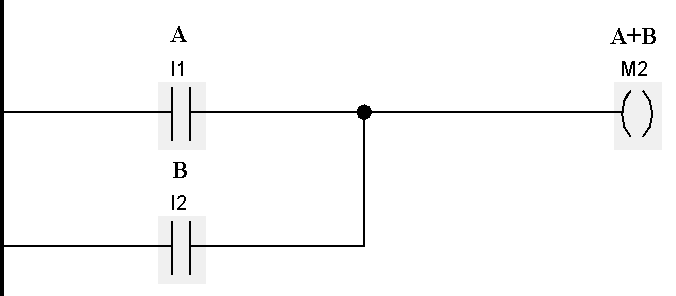NETWORK 2 :-

Here combinational logic is used for commutative laws. Final result will be Q1.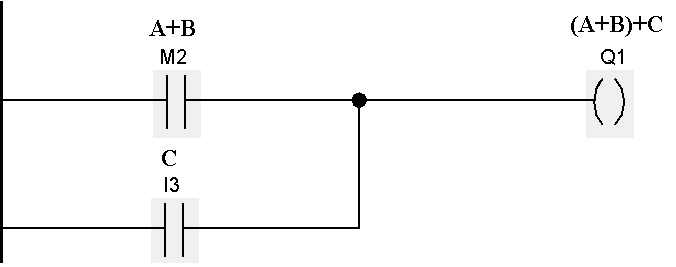NETWORK 3 :-

Here AND gate is used for inputs A and B. Result will be stored in M1 coil.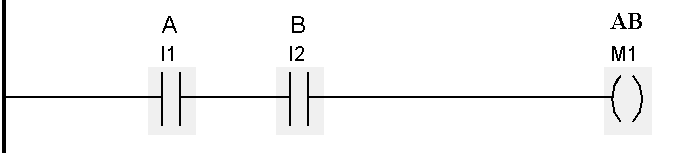NETWORK 4 :-

Combinational logic is used for associate law. Final result will be Q2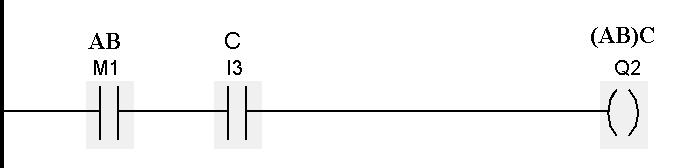NETWORK 5 :-

AND gate of two inputs (A&B) for distributive law. Result will be stored in M3.NETWORK 6 :-

AND gate of two inputs (A&C) for distributive law. Result will be stored in M4.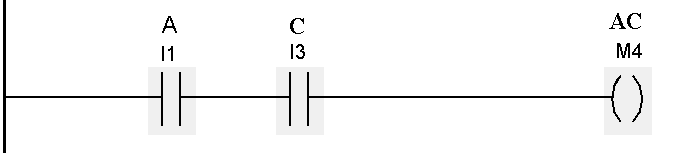NETWORK 7 :-

OR gate of M3 and M4. Result will be stored in Q3.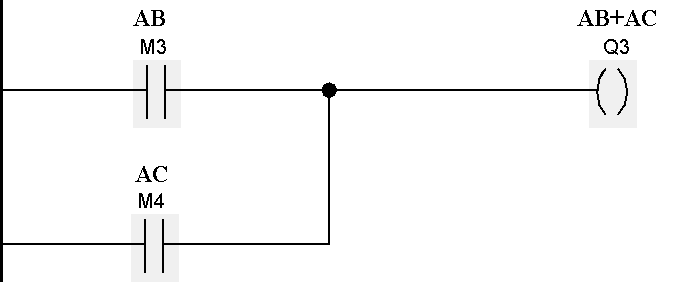NETWORK 8 :-

Other combination of distributive law.Note:-Above application may be different from actual application. This example is only for explanation and educational purpose only. We can implement this logic in other PLC also. This is the simple concept for Laws of Boolean algebra using ladder logic, we can use this concept in other examples also.

All parameters and graphical representations considered in this example are for explanation purpose only, parameters or representation may be different in actual applications. Also all interlocks are not considered in the application.

Articles You May Like :

Boolean Algebraic Identities

PLC Input Output Modules

Introduction to Karnaugh Mapping

What is Kirchhoff’s Current Law

PLC Ladder Logic for Lube Oil Pump Motor

This website uses cookies to improve your experience. We'll assume you're ok with this, but you can opt-out if you wish. Accept Read More

WordPress Image Lightbox

Send this to a friend